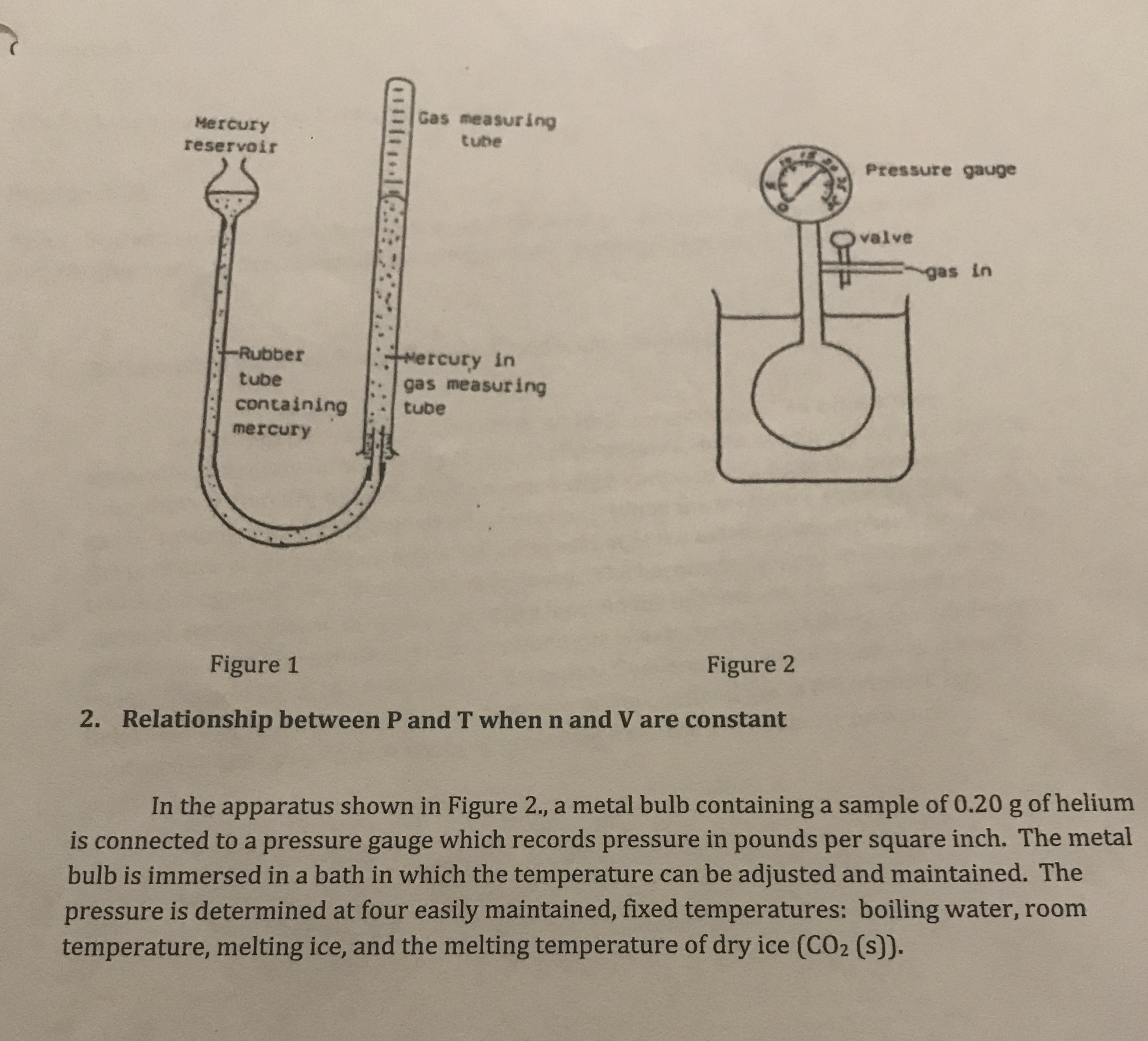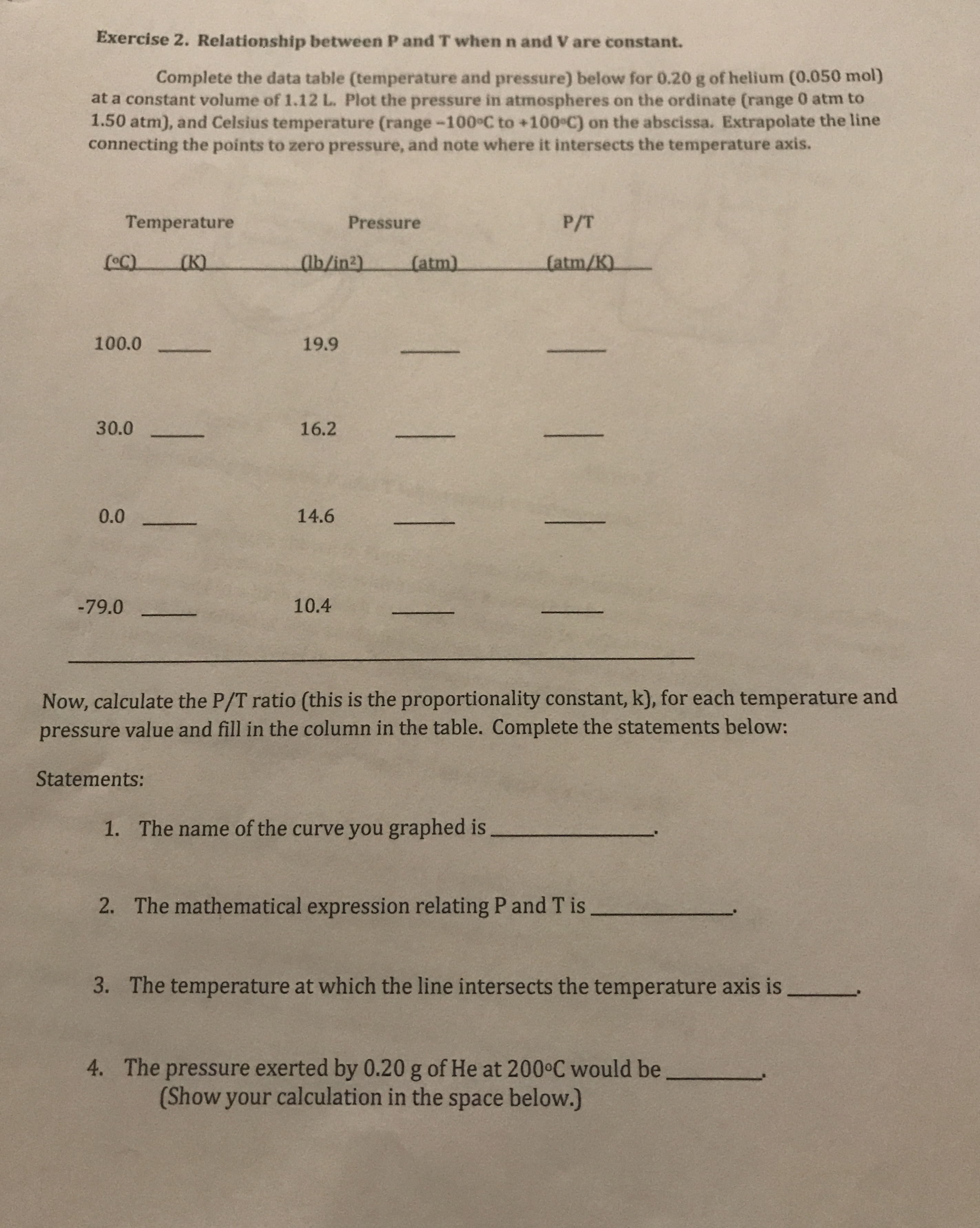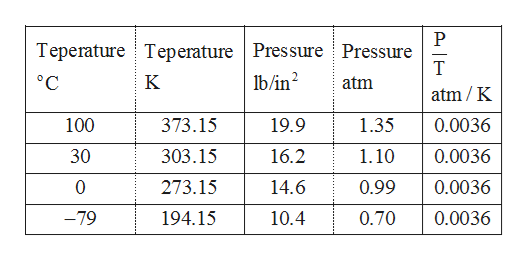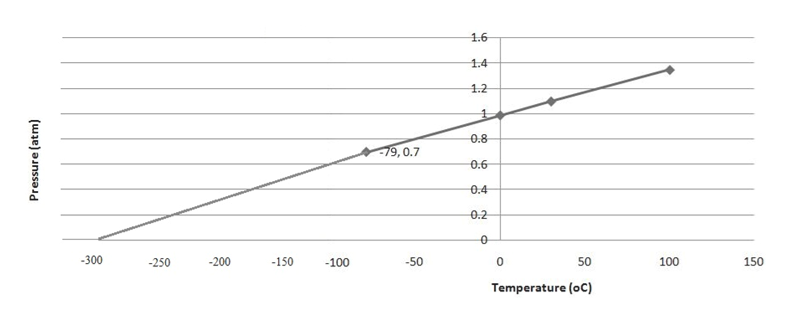# Gas measuringMercurytubereservoirPressure gaugevalvegas inRubberMercury intubegas measuringcontainingtubemercuryFigure 1Figure 2Relationship between P and T when n and V are constant2.In the apparatus shown in Figure 2., a metal bulb containing a sample of 0.20 g of heliumis connected to a pressure gauge which records pressure in pounds per square inch. The metalbulb is immersed in a bath in which the temperature can be adjusted and maintained. Thepressure is determined at four easily maintained, fixed temperatures: boiling water, roomtemperature, melting ice, and the melting temperature of dry ice (CO2 (s)). Exercise 2. Relationship between P and T when n and V are constant.Complete the data table (temperature and pressure) below for 0.20 g of helium (0.050 mol)at a constant volume of 1.12 L. Plot the pressure in atmospheres on the ordinate (range 0 atm to1.50 atm), and Celsius temperature (range-100°C to +100 C) on the abscissa. Extrapolate the lineconnecting the points to zero pressure, and note where it intersects the temperature axis.TemperatureP/TPressure(atm/K)(K)ab/in2)(atm)100.019.930.016.214.60.010.4-79.0Now, calculate the P/T ratio (this is the proportionality constant, k), for each temperature andpressure value and fill in the column in the table. Complete the statements below:Statements:The name of the curve you graphed is1.The mathematical expression relating P and T is2.The temperature at which the line intersects the temperature axis is3.The pressure exerted by 0.20 g of He at 200 C would be(Show your calculation in the space below.)4.| ! 1 1

Questionhelp_outlineImage TranscriptioncloseGas measuring Mercury tube reservoir Pressure gauge valve gas in Rubber M ercury in tube gas measuring containing tube mercury Figure 1 Figure 2 Relationship between P and T when n and V are constant 2. In the apparatus shown in Figure 2., a metal bulb containing a sample of 0.20 g of helium is connected to a pressure gauge which records pressure in pounds per square inch. The metal bulb is immersed in a bath in which the temperature can be adjusted and maintained. The pressure is determined at four easily maintained, fixed temperatures: boiling water, room temperature, melting ice, and the melting temperature of dry ice (CO2 (s)). fullscreenhelp_outlineImage TranscriptioncloseExercise 2. Relationship between P and T when n and V are constant. Complete the data table (temperature and pressure) below for 0.20 g of helium (0.050 mol) at a constant volume of 1.12 L. Plot the pressure in atmospheres on the ordinate (range 0 atm to 1.50 atm), and Celsius temperature (range-100°C to +100 C) on the abscissa. Extrapolate the line connecting the points to zero pressure, and note where it intersects the temperature axis. Temperature P/T Pressure (atm/K) (K) ab/in2) (atm) 100.0 19.9 30.0 16.2 14.6 0.0 10.4 -79.0 Now, calculate the P/T ratio (this is the proportionality constant, k), for each temperature and pressure value and fill in the column in the table. Complete the statements below: Statements: The name of the curve you graphed is 1. The mathematical expression relating P and T is 2. The temperature at which the line intersects the temperature axis is 3. The pressure exerted by 0.20 g of He at 200 C would be (Show your calculation in the space below.) 4. | ! 1 1 fullscreen
check_circleExpert Solution
Step 1

Unit conversion is given below.

Kelvin scale = oC +273.15

1 lb/in2 = 1/14.696 atmhelp_outlineImage TranscriptioncloseTeperature Teperature Pressure Pressure lb/in T °C K atm atm/K 100 373.15 19.9 1.35 0.0036 303.15 1.10 30 16.2 0.0036 0.0036 273.15 14.6 0.99 -79 194.15 10.4 0.70 0.0036 fullscreen
Step 2

Pressure-Temperature grap...help_outlineImage Transcriptionclose1.6 1.4 1.2 1 0.8 79,0.7 0.6 0.4 0.2 -300 -200 150 -50 100 150 -250 -100 Temperature (oC) Pressure (atm) 50 fullscreen

### Want to see the full answer?

See Solution

#### Want to see this answer and more?

Solutions are written by subject experts who are available 24/7. Questions are typically answered within 1 hour*

See Solution
*Response times may vary by subject and question
Tagged in

### Chemistry